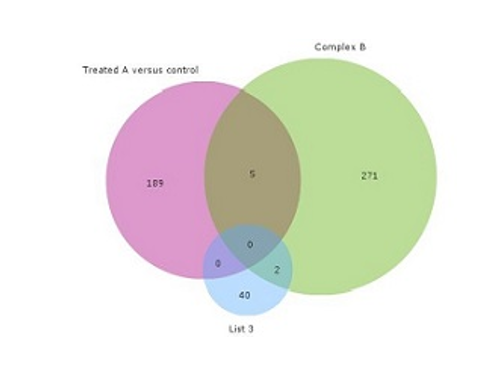# Introduction to the Venn diagram plot and the Set tool

Introduction to the Venn diagram in Qlucore Omics Explorer as an excellent tool to visualize overlap and interaction between lists. It is easy to set up and configure the plot. In combination with the Set tool you can both visualize and export your results as lists.

Category: Introduction

Short introduction video

Watch here

Get started now with a free 10 days trial of Qlucore Omics Explorer!

Start here